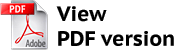https://vestnik.volbi.ru/
﻿

# A QUASI-PROBABILISTIC MODEL OF THE COST PRICE CALCULATION

Back to articles of current journal
 PDF:Author(s): Yatsko V. A., Yudina L. N., Number of journal: 2(43) Date: May 2018 Annotation: The problem of ambiguity in calculating the cost price is examined. A brief analysis of the known approaches to the cost price calculation is given. It is shown that the main problem of costing is the incorrect distribution of indirect costs per units. In order to describe the uncertainty inherent to the problem of indirect cost allocation, it is proposed to use an approach based on the construction of quasi-probabilistic models (in particular, random variables). An analytical expression was first obtained for the distribution density of quasi-random variable characterizing the possible cost values. Keywords: calculation, cost price, full prime cost, indirect costs, indirect costs allocation, costs driver, quasi-probabilistic models, random variable, distribution density, hypothesis testing. For citation: Yudina L. N., Yatsko V. A. A quasi-probabilistic model of the cost prise calculation // Business. Education. Law. 2018. No. 2 (43). P. 225–228. DOI: 10.25683/VOLBI.2018.43.245.